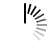# Algorithms and Complexities

#### Algorithm

An algorithm is a finite set of instructions, those if followed, accomplishes a particular task. It is not language specific, we can use any language and symbols to represent instructions.

#### The criteria of an algorithm

1. Input: Zero or more inputs are externally supplied to the algorithm.
2. Output: At least one output is produced by an algorithm.
3. Definiteness: Each instruction is clear and unambiguous.
4. Finiteness: In an algorithm, it will be terminated after a finite number of steps for all different cases.
5. Effectiveness: Each instruction must be very basic, so the purpose of those instructions must be very clear to us.

#### Analysis of algorithms

Algorithm analysis is an important part of computational complexities. The complexity theory provides the theoretical estimates for the resources needed by an algorithm to solve any computational task. Analysis of the algorithm is the process of analyzing the problem-solving capability of the algorithm in terms of the time and size required (the size of memory for storage while implementation). However, the main concern of the analysis of the algorithm is the required time or performance.

#### Complexities of an Algorithm

The complexity of an algorithm computes the amount of time and spaces required by an algorithm for an input of size (n). The complexity of an algorithm can be divided into two types. The time complexity and the space complexity.

#### Time Complexity of an Algorithm

The time complexity is defined as the process of determining a formula for total time required towards the execution of that algorithm. This calculation is totally independent of implementation and programming language.

#### Space Complexity of an Algorithm

Space complexity is defining as the process of defining a formula for prediction of how much memory space is required for the successful execution of the algorithm. The memory space is generally considered as the primary memory.